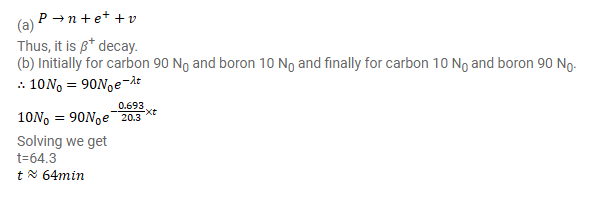# Carbon (Z=6) with mass number 11 decays to boron (Z=5).

Question:

Carbon $(Z=6)$ with mass number 11 decays to boron $(Z=5)$.

(a) Is it a $\beta^{+}$-decay or a $\beta^{-}$decay?

(b) The half-life of the decay scheme is $20.3$ minutes. How much time will elapse before a mixture of $90 \%$ carbon-11 and $10 \%$ boron-11 (by the number of atoms) converts itself into a mixture of $10 \%$ carbon- 11 and $90 \%$ boron- 11 ?

Solution: